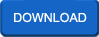## Resource Management Techniques

 Linear Programming: IntroductionLinear programming is the process of taking various linear inequalities relating to some situation, and finding the "best" value obtainable under those conditions. A typical example would be taking the limitations of materials and labor, and then determining the "best" production levels for maximal profits under those conditions.
Rate this Book:
 The Critical Path MethodBackground,Some questions about the project,What is the Critical Path Method(CPM)?,Why the CPM? How to use the CPM ?,Questions and Answers
Rate this Book:
 Transportation, Assignment and Transshipment ProblemsA transportation problem basically deals with the problem, which aims to find the best way to fulfill the demand of n demand points using the capacities of m supply points.
Rate this Book:
 Senstive AnalysisWe have already been introduced to sensitivity analysis in Chapter 1 via the geometry of a simple example. We saw that the values of the decision variables and those of the slack and surplus variables remain unchanged even though some coefficients in the objective function are varied.
Rate this Book:
 The Dual Linear ProgramIn discussing duality, it is common to depart from the standard equality form of the LP given in Section 4.1 in order to highlight the symmetry of the primal-dual relationships.
Rate this Book:
 Resource levelingOver allocation means the scheduled work is not likely to occur (or the resource may be annoyed, overworked or burned out). Under allocation means suboptimal utilizing and billing of resource, lowering of profitability. Lastly, your schedule won’t be accurate, unless resources are correctly loaded.
Rate this Book:
 Project Network DiagramProject Network Diagram are explained in this book
Rate this Book:
 PERT for Project Planning and SchedulingPERT, the project Evaluation and Review Technique, is a network-based aid for planning and scheduling the many interrelated tasks in a large and complex project
Rate this Book:
 Classical Optimization TheoryPreliminaries,Necessary Conditions for Constrained Local Maximum and Minimum,Sufficient Conditions for Constrained Local Maximum and Minimum,etc..,
Rate this Book:
 Nonlinear Programming and the Kuhn-Tucker ConditionsOur constrained optimization analysis has dealt only with situations in which there is a single constraint, and in which all the variables are generally positive at the optimum.
Rate this Book:
 Linear ProgrammingDuality,Pivot Operation,Simple Method,Generalized Duality,Cycling,etc..,
Rate this Book:
 Linear Programming ModelsSteps in Developing a Linear Programming (LP) Model,Properties of LP Models,Example LP Model Formulation: The Product Mix Problem, Example: Flair Furniture Co,etc..,
Rate this Book:
 Lagrangian MethodsLagrangian Methods and example are been expalined in this book
Rate this Book:
 Kuhn-Tucker conditionsIn this document, we set out the constrained optimisation with inequality constraints and state the Kuhn-Tucker necessary conditions for a solution; after an example, we state the Kuhn-Tucker sufficient conditions for a maximum.
Rate this Book:
 Integer Programming - BasicsThe linear-programming models that have been discussed thus far all have been continuous, in the sense that decision variables are allowed to be fractional.
Rate this Book:
 Integer ProgrammingMixed and Pure Integer Programming Problems,Examples,Further Uses of Integer Variables,Solving IP Problems: Cutting Plane Algorithm etc..,
Rate this Book:
 Dual Simplex AlgorithmDual Simplex Algorithm and the definition are explained in this chapter
Rate this Book:
 The Dual Simplex MethodAlgorithm as explained so far known as primal simplex,starting with feasible basis, look for optimal basis while keeping feasibility.Alternative algorithm known as dual simplex,starting with optimal basis, look for feasible basis while keeping optimality
Rate this Book:
 Duality and Sensitivity AnalysisThe optimal solution of a linear-programming problem represents a snapshot of the conditions that prevail at the time of the model is formulated
Rate this Book:
 Decision Making and Problem SolvingDecision making and problem solving are critically important skill areas for emergency managers, planners, first responders, voluntary agency coordinators, and other professionals in emergency management.
Rate this Book: